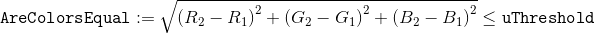﻿ Threshold Property (CompareBitmapCommand) | Leadtools.ImageProcessing.Core | Raster, Medical, Document Help
←Select platform
In This Topic ▼

# Threshold Property

Summary

An unsigned integer specifying the equality threshold for colors.

Syntax
C#
Objective-C
C++/CLI
Java
Python
``public uint Threshold {get; set;} ``
``@property (nonatomic, assign) NSUInteger threshold; ``
````public long getThreshold(); `
`public void setThreshold( `
`   long longValue `
`); ````
````public:  `
`   property UInt32 Threshold `
`   { `
`      UInt32 get() `
`      void set(UInt32 value) `
`   } ````
``Threshold # get and set (CompareBitmapCommand) ``

#### Property Value

The maximum distance between two colors that can still be considered to be equal. The default value is 0.

Remarks

The Threshold defines the range in color values allowed and still be considered the same color. The distance is calculated in Euclidean RGB color space using the following equation:Example
C#
````using Leadtools; `
`using Leadtools.Codecs; `
`using Leadtools.ImageProcessing; `
`using Leadtools.ImageProcessing.Core; `
` `
`public void CompareBitmapCommandExample() `
`{ `
`   using (RasterCodecs codecs = new RasterCodecs()) `
`   // Load the original image `
`   using (RasterImage referenceImage = codecs.Load(Path.Combine(LEAD_VARS.ImagesDir, "ocr1.tif"))) `
`   // Use the same image for the "modified" image `
`   using (RasterImage modifiedImage = referenceImage.Clone()) `
`   { `
`      // Remove the last paragraph of the reference image `
`      referenceImage.AddRectangleToRegion(null, new LeadRect(290, 2470, 1930, 360), RasterRegionCombineMode.Set); `
`      new FillCommand(RasterColor.White).Run(referenceImage); `
`      referenceImage.MakeRegionEmpty(); `
` `
`      // Remove the title from the modified image `
`      modifiedImage.AddRectangleToRegion(null, new LeadRect(290, 300, 810, 110), RasterRegionCombineMode.Set); `
`      new FillCommand(RasterColor.White).Run(modifiedImage); `
`      modifiedImage.MakeRegionEmpty(); `
` `
`      // Rotate the modified image for demonstration (angle measured in hundredths of a degree) `
`      new RotateCommand(340 * 100, RotateCommandFlags.Resize, RasterColor.Black).Run(modifiedImage); `
` `
`      // Update the transformation to align/reverse the above rotation `
`      LeadMatrix alignment = LeadMatrix.Identity; `
`      alignment.Translate(-modifiedImage.Width * 0.5, -modifiedImage.Height * 0.5); `
`      alignment.Rotate(20.0); `
`      alignment.Translate(referenceImage.Width * 0.5, referenceImage.Height * 0.5); `
` `
`      // Setup the comparison options `
`      CompareBitmapCommand command = new CompareBitmapCommand() `
`      { `
`         Alignment = alignment, `
`         ReferenceImage = referenceImage `
`      }; `
` `
`      // Compare the images `
`      command.Run(modifiedImage); `
` `
`      // Save the results `
`      using (RasterImage outputImage = command.OutputImage) `
`         codecs.Save(outputImage, Path.Combine(LEAD_VARS.ImagesDir, "CompareBitmap_Output.png"), RasterImageFormat.Png, 0); `
` `
`      // Save the two input images, for reference `
`      codecs.Save(referenceImage, Path.Combine(LEAD_VARS.ImagesDir, "CompareBitmap_Reference.png"), RasterImageFormat.Png, 0); `
`      codecs.Save(modifiedImage, Path.Combine(LEAD_VARS.ImagesDir, "CompareBitmap_Modified.png"), RasterImageFormat.Png, 0); `
`   } `
`} `
` `
`static class LEAD_VARS `
`{ `
`   public const string ImagesDir = @"C:\LEADTOOLS22\Resources\Images"; `
`} ````
Requirements